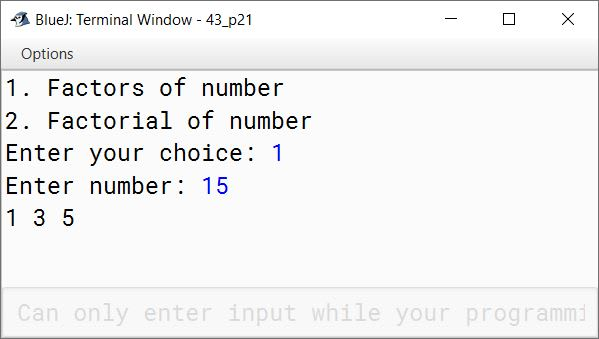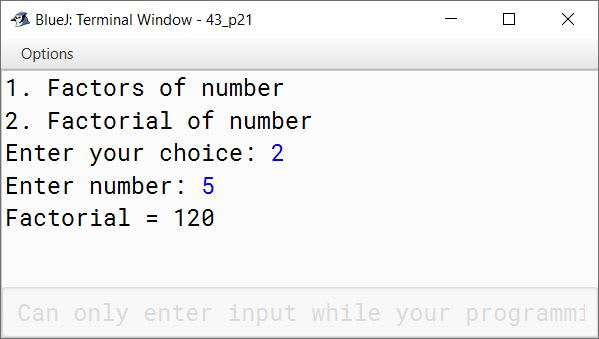# Solved 2015 Question Paper ICSE Class 10 Computer Applications

## Section A

#### Question 1

(a) What are the default values of the primitive data type int and float?

Default value of int is 0 and float is 0.0f.

(b) Name any two OOP’s principles.

1. Encapsulation
2. Inheritance

(c) What are identifiers ?

Identifiers are symbolic names given to different parts of a program such as variables, methods, classes, objects, etc.

(d) Identify the literals listed below:

(i) 0.5 (ii) 'A' (iii) false (iv) "a"

1. Real Literal
2. Character Literal
3. Boolean Literal
4. String Literal

(e) Name the wrapper classes of char type and boolean type.

Wrapper class of char type is Character and boolean type is Boolean.

#### Question 2

(a) Evaluate the value of n if value of p = 5, q = 19

int n = (q – p) > (p – q) ? (q – p) : (p – q);

int n = (q – p) > (p – q) ? (q – p) : (p – q)
⇒ int n = (19 - 5) > (5 - 19) ? (19 - 5) : (5 - 19)
⇒ int n = 14 > -14 ? 14 : -14
⇒ int n = 14 [∵ 14 > -14 is true so ? : returns 14]

Final value of n is 14

(b) Arrange the following primitive data types in an ascending order of their size:

(i) char (ii) byte (iii) double (iv) int

byte, char, int, double

(c) What is the value stored in variable res given below:

double res = Math.pow ("345".indexOf('5'), 3);

Value of res is 8.0
Index of '5' in "345" is 2. Math.pow(2, 3) means 23 which is equal to 8

(d) Name the two types of constructors

Parameterized constructors and Non-Parameterized constructors.

(e) What are the values of a and b after the following function is executed, if the values passed are 30 and 50:

void paws(int a, int b)
{
a=a+b;
b=a–b;
a=a–b;
System.out.println(a+ "," +b);
}

Value of a is 50 and b is 30.
Output of the code is:

50,30


#### Question 3

(a) State the data type and value of y after the following is executed:

char x='7';
y=Character.isLetter(x);

Data type of y is boolean and value is false. Character.isLetter() method checks if its argument is a letter or not and as 7 is a number not a letter hence it returns false.

(b) What is the function of catch block in exception handling ? Where does it appear in a program ?

Catch block contains statements that we want to execute in case an exception is thrown in the try block. Catch block appears immediately after the try block in a program.

(c) State the output when the following program segment is executed:

String a ="Smartphone", b="Graphic Art";
String h=a.substring(2, 5);
String k=b.substring(8).toUpperCase();
System.out.println(h);
System.out.println(k.equalsIgnoreCase(h));

The output of the above code is:

art
true

##### Explanation

a.substring(2, 5) extracts the characters of string a starting from index 2 till index 4. So h contains the string "art". b.substring(8) extracts characters of b from index 8 till the end so it returns "Art". toUpperCase() converts it into uppercase. Thus string "ART" gets assigned to k. Values of h and k are "art" and "ART", respectively. As both h and k differ in case only hence equalsIgnoreCase returns true.

(d) The access specifier that gives the most accessibility is ________ and the least accessibility is ________.

The access specifier that gives the most accessibility is public and the least accessibility is private.

(e) (i) Name the mathematical function which is used to find sine of an angle given in radians.
(ii) Name a string function which removes the blank spaces provided in the prefix and suffix of a string.

(i) Math.sin() (ii) trim()

(f) (i) What will this code print ?

int arr[]=new int;
System.out.println(arr);
1. 0
2. value stored in arr
3. 0000
4. garbage value

This code will print garbage value

(ii) Name the keyword which is used- to resolve the conflict between method parameter and instance variables/fields.

this keyword

(g) State the package that contains the class:

2. Scanner

1. java.io
2. java.util

(h) Write the output of the following program code:

char ch ;
int x=97;
do
{
ch=(char)x;
System.out.print(ch + " " );
if(x%10 == 0)
break;
++x;
} while(x<=100);

The output of the above code is:

a b c d

##### Explanation

The below table shows the dry run of the program:

xchOutputRemarks
97aa1st Iteration
98ba b2nd Iteration
99ca b c3rd Iteration
100da b c d4th Iteration — As x%10 becomes true, break statement is executed and exists the loop.

(i) Write the Java expressions for:

$\dfrac{a^2+b^2}{2ab}$

(a * a + b * b) / (2 * a * b)

(j) If int y = 10 then find int z = (++y * (y++ + 5));

z = (++y * (y++ + 5))
⇒ z = (11 * (11 + 5))
⇒ z = (11 * 16)
⇒ z = 176

## Section B

#### Question 4

Define a class called ParkingLot with the following description:

Instance variables/data members:
int vno — To store the vehicle number.
int hours — To store the number of hours the vehicle is parked in the parking lot.
double bill — To store the bill amount.

Member Methods:
void input() — To input and store the vno and hours.
void calculate() — To compute the parking charge at the rate of ₹3 for the first hour or part thereof, and ₹1.50 for each additional hour or part thereof.
void display() — To display the detail.

Write a main() method to create an object of the class and call the above methods.

import java.util.Scanner;

public class ParkingLot
{
private int vno;
private int hours;
private double bill;

public void input() {
Scanner in = new Scanner(System.in);
System.out.print("Enter vehicle number: ");
vno = in.nextInt();
System.out.print("Enter hours: ");
hours = in.nextInt();
}

public void calculate() {
if (hours <= 1)
bill = 3;
else
bill = 3 + (hours - 1) * 1.5;
}

public void display() {
System.out.println("Vehicle number: " + vno);
System.out.println("Hours: " + hours);
System.out.println("Bill: " + bill);
}

public static void main(String args[]) {
ParkingLot obj = new ParkingLot();
obj.input();
obj.calculate();
obj.display();
}
}
##### Output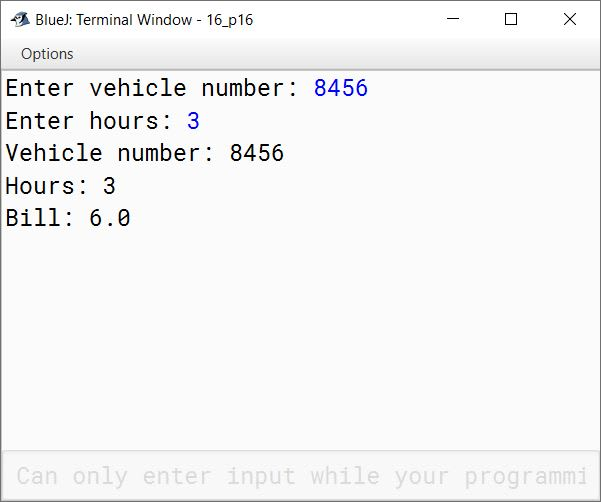#### Question 5

Write two separate programs to generate the following patterns using iteration (loop) statements:

(a)

*
*  #
*  #  *
*  #  *  #
*  #  *  #  *

(b)

5 4 3 2 1
5 4 3 2
5 4 3
5 4
5

public class KboatPattern
{
public static void main(String args[]) {
for (int i = 1; i <=5; i++) {
for (int j = 1; j <= i; j++) {
if (j % 2 == 0)
System.out.print("# ");
else
System.out.print("* ");
}
System.out.println();
}
}
}
##### Output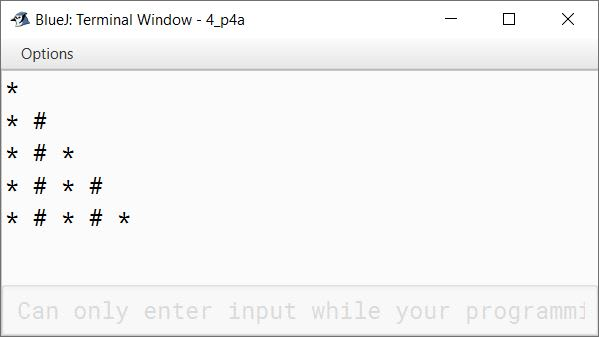public class KboatPattern
{
public static void main(String args[]) {
for (int i = 1; i <=5; i++) {
for (int j = 5; j >= i; j--) {
System.out.print(j + " ");
}
System.out.println();
}
}
}
##### Output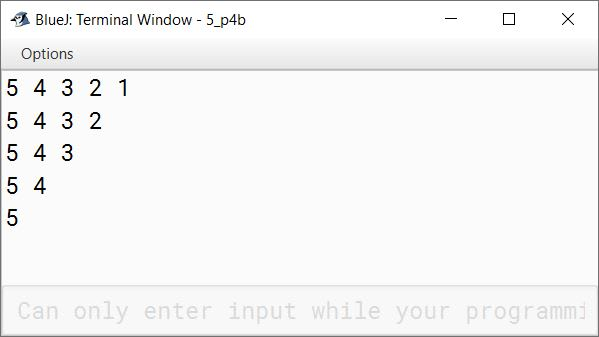#### Question 6

Write a program to input and store roll numbers, names and marks in 3 subjects of n number of students in five single dimensional arrays and display the remark based on average marks as given below:

Average MarksRemark
85 — 100Excellent
75 — 84Distinction
60 — 74First Class
40 — 59Pass
Less than 40Poor

import java.util.Scanner;

public class KboatAvgMarks
{
public static void main(String args[]) {

Scanner in = new Scanner(System.in);
System.out.print("Enter number of students: ");
int n = in.nextInt();

int rollNo[] = new int[n];
String name[] = new String[n];
int s1[] = new int[n];
int s2[] = new int[n];
int s3[] = new int[n];
double avg[] = new double[n];

for (int i = 0; i < n; i++) {
System.out.println("Enter student " + (i+1) + " details:");
System.out.print("Roll No: ");
rollNo[i] = in.nextInt();
in.nextLine();
System.out.print("Name: ");
name[i] = in.nextLine();
System.out.print("Subject 1 Marks: ");
s1[i] = in.nextInt();
System.out.print("Subject 2 Marks: ");
s2[i] = in.nextInt();
System.out.print("Subject 3 Marks: ");
s3[i] = in.nextInt();
avg[i] = (s1[i] + s2[i] + s3[i]) / 3.0;
}

System.out.println("Roll No\tName\tRemark");
for (int i = 0; i < n; i++) {
String remark;
if (avg[i] < 40)
remark = "Poor";
else if (avg[i] < 60)
remark = "Pass";
else if (avg[i] < 75)
remark = "First Class";
else if (avg[i] < 85)
remark = "Distinction";
else
remark = "Excellent";
System.out.println(rollNo[i] + "\t"
+ name[i] + "\t"
+ remark);
}
}
}
##### Output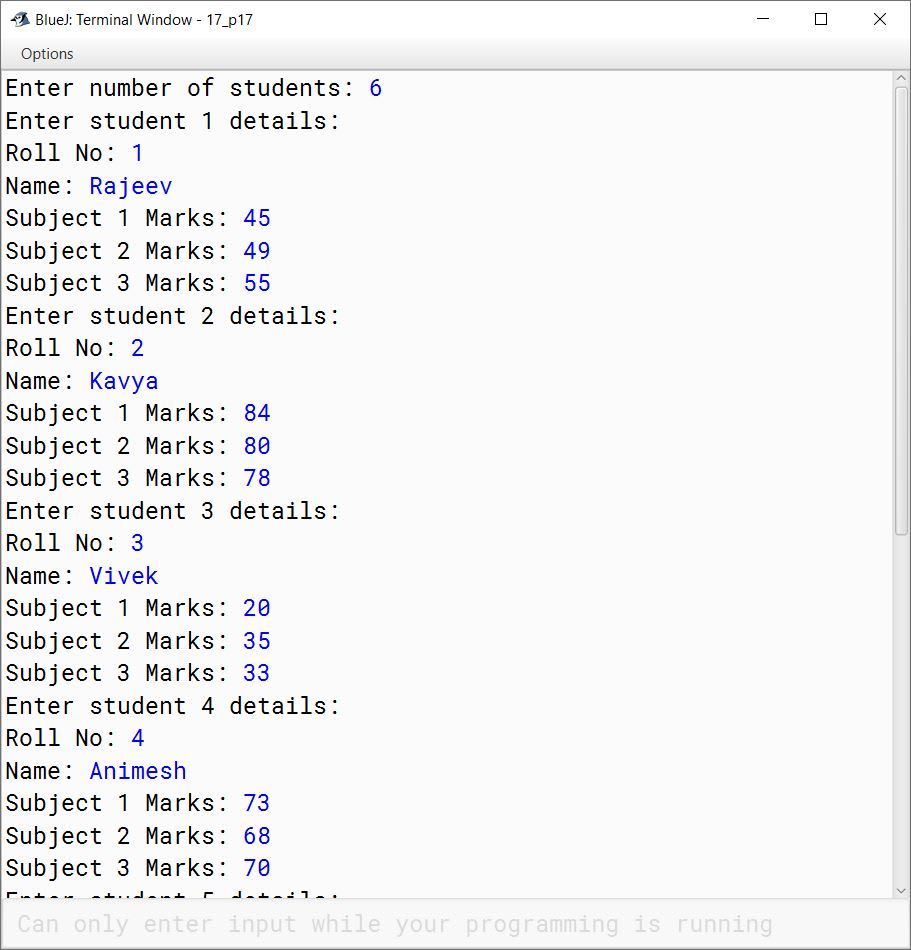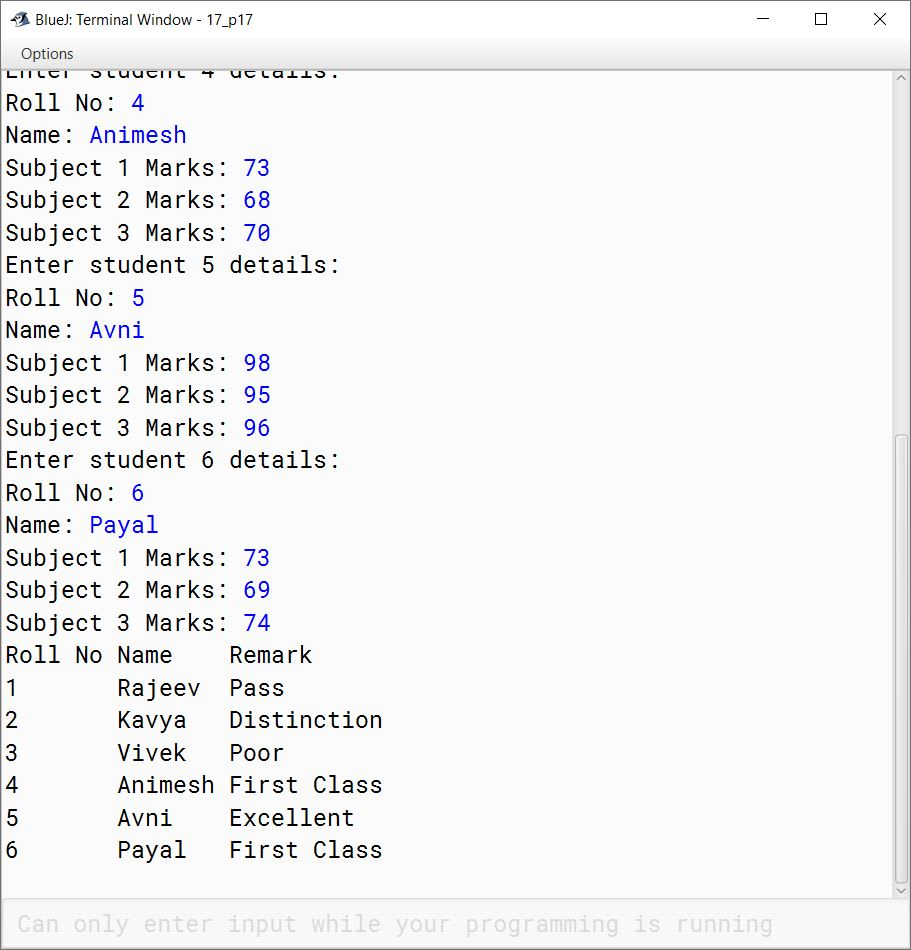#### Question 7

Design a class to overload a function Joystring( ) as follows:

1. void Joystring(String s, char ch1, char ch2) with one string argument and two character arguments that replaces the character argument ch1 with the character argument ch2 in the given String s and prints the new string.
Example:
Input value of s = "TECHNALAGY"
ch1 = 'A'
ch2 = 'O'
Output: "TECHNOLOGY"
2. void Joystring(String s) with one string argument that prints the position of the first space and the last space of the given String s.
Example:
Input value of s = "Cloud computing means Internet based computing"
Output:
First index: 5
Last Index: 36
3. void Joystring(String s1, String s2) with two string arguments that combines the two strings with a space between them and prints the resultant string.
Example:
Input value of s1 = "COMMON WEALTH"
Input value of s2 = "GAMES"
Output: COMMON WEALTH GAMES

(Use library functions)

public class KboatStringOverload
{
public void joystring(String s, char ch1, char ch2) {
String newStr = s.replace(ch1, ch2);
System.out.println(newStr);
}

public void joystring(String s) {
int f = s.indexOf(' ');
int l = s.lastIndexOf(' ');
System.out.println("First index:  " + f);
System.out.println("Last index:  " + l);
}

public void joystring(String s1, String s2) {
String newStr = s1.concat(" ").concat(s2);
System.out.println(newStr);
}

public static void main(String args[]) {
obj.joystring("TECHNALAGY", 'A', 'O');
obj.joystring("Cloud computing means Internet based computing");
obj.joystring("COMMON WEALTH", "GAMES");
}
}
##### Output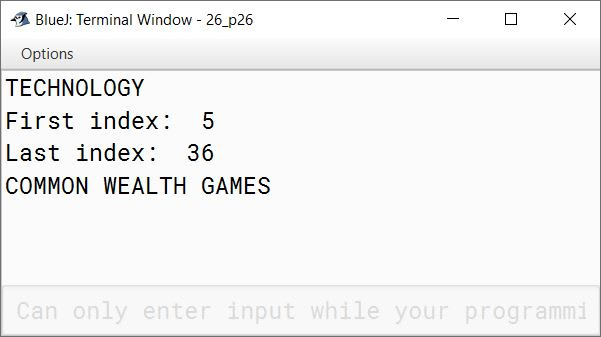#### Question 8

Write a program to input twenty names in an array. Arrange these names in descending order of letters, using the bubble sort technique.

import java.util.Scanner;

public class KboatArrangeNames
{
public static void main(String args[]) {
Scanner in = new Scanner(System.in);
String names[] = new String;
System.out.println("Enter 20 names:");
for (int i = 0;  i < names.length; i++) {
names[i] = in.nextLine();
}

//Bubble Sort
for (int i = 0; i < names.length - 1; i++) {
for (int j = 0; j < names.length - 1 - i; j++) {
if (names[j].compareToIgnoreCase(names[j + 1]) < 0) {
String temp = names[j + 1];
names[j + 1] = names[j];
names[j] = temp;
}
}
}

System.out.println("\nSorted Names");
for (int i = 0;  i < names.length; i++) {
System.out.println(names[i]);
}
}
}
##### Output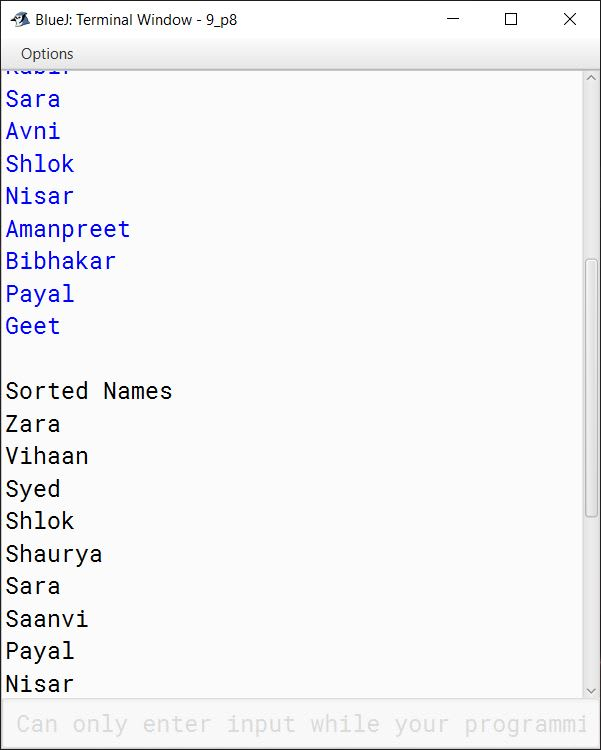#### Question 9

Using switch statement, write a menu driven program to:
(i) To find and display all the factors of a number input by the user ( including 1 and the excluding the number itself).
Example:
Sample Input : n = 15
Sample Output : 1, 3, 5

(ii) To find and display the factorial of a number input by the user (the factorial of a non-negative integer n, denoted by n!, is the product of all integers less than or equal to n.)
Example:
Sample Input : n = 5
Sample Output : 5! = 1*2*3*4*5 = 120

For an incorrect choice, an appropriate error message should be displayed.

import java.util.Scanner;

{
public static void main(String args[]) {
Scanner in = new Scanner(System.in);
System.out.println("1. Factors of number");
System.out.println("2. Factorial of number");
int choice = in.nextInt();
int num;

switch (choice) {
case 1:
System.out.print("Enter number: ");
num = in.nextInt();
for (int i = 1; i < num; i++) {
if (num % i == 0) {
System.out.print(i + " ");
}
}
System.out.println();
break;

case 2:
System.out.print("Enter number: ");
num = in.nextInt();
int f = 1;
for (int i = 1; i <= num; i++)
f *= i;
System.out.println("Factorial = " + f);
break;

default:
System.out.println("Incorrect Choice");
break;
}
}
}
##### Output